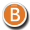#### Hair

• 3D Figure Assets 3D Models

By:  hameleon ()

34.96 USD
30% off• 3D Figure Assets 3D Models

By:  hameleon ()

34.96 USD
30% off• 3D Models

By:  RPublishing (),  GrayCloudDesign ()

13.96 USD
30% off• 3D Figure Assets

By:  -Wolfie- ()

6.96 USD
30% off• 3D Figure Assets

By:  -Wolfie- ()

6.96 USD
30% off• 3D Figure Assets

By:  -Wolfie- ()

6.96 USD
30% off• 3D Figure Assets

By:  -Wolfie- ()

6.96 USD
30% off• 3D Figure Assets

By:  LUNA3D ()

9.71 USD
25% off• 3D Figure Assets

By:  -Wolfie- ()

9.95 USD• 3D Figure Assets

By:  -Wolfie- ()

9.95 USD• 3D Figure Assets

By:  Dream9Studios ()

9.99 USD• 3D Figure Assets

By:  -Wolfie- ()

9.95 USD• 3D Figure Assets

By:  -Wolfie- ()

9.95 USD• 3D Figure Assets

By:  -Wolfie- ()

9.95 USD• 3D Figure Assets

By:  -Wolfie- ()

9.95 USD• 3D Figure Assets

By:  -Wolfie- ()

9.95 USD• 3D Figure Assets

By:  -Wolfie- ()

9.95 USD• 3D Figure Assets

By:  -Wolfie- ()

9.95 USD• 3D Figure Assets

By:  -Wolfie- ()

9.95 USD• 3D Figure Assets

By:  -Wolfie- ()

5.00 USD
50% off• 3D Figure Assets

By:  -Wolfie- ()

9.95 USD• 3D Figure Assets

By:  -Wolfie- ()

9.95 USD• 3D Figure Assets

By:  -Wolfie- ()

9.95 USD• 3D Figure Assets

By:  -Wolfie- ()

9.95 USD• 3D Figure Assets

By:  -Wolfie- ()

9.95 USD• 3D Figure Assets La Femme - LHomme Poser Figures

By:  -Wolfie- ()

9.95 USD• 3D Figure Assets

By:  -Wolfie- ()

9.95 USD• 3D Figure Assets

By:  -Wolfie- ()

9.95 USD• 3D Figure Assets

By:  LUNA3D ()

9.06 USD
30% off• 3D Figure Assets

By:  -Wolfie- ()

9.95 USD• 3D Figure Assets

By:  -Wolfie- ()

9.95 USD• 3D Figure Assets

By:  -Wolfie- ()

9.95 USD• 3D Figure Assets

By:  3DTubeMagic ()

9.95 USD• 3D Figure Assets

By:  -Wolfie- ()

5.00 USD
50% off• 3D Figure Assets

By:  -Wolfie- ()

9.95 USD• 3D Figure Assets

By:  -Wolfie- ()

9.95 USD• 3D Figure Assets

By:  -Wolfie- ()

9.95 USD• 3D Figure Assets

By:  -Wolfie- ()

9.95 USD• 3D Figure Assets La Femme - LHomme Poser Figures

By:  -Wolfie- ()

5.00 USD
50% off• 3D Figure Assets

By:  -Wolfie- ()

9.95 USD• 3D Figure Assets

By:  -Wolfie- ()

9.95 USD• 3D Figure Assets

By:  -Wolfie- ()

9.95 USD• 3D Figure Assets

By:  -Wolfie- ()

9.95 USD• 3D Figure Assets

By:  -Wolfie- ()

9.95 USD• 3D Figure Assets

By:  -Wolfie- ()

9.95 USD• 3D Figure Assets

By:  -Wolfie- ()

9.95 USD• 3D Figure Assets

By:  -Wolfie- ()

9.95 USD• 3D Figure Assets

By:  -Wolfie- ()

9.95 USD• 3D Figure Assets

By:  -Wolfie- ()

9.95 USD• 3D Figure Assets

By:  -Wolfie- ()

9.95 USD• 3D Figure Assets

By:  -Wolfie- ()

9.95 USD